# RD Sharma Solutions for Class 10 Maths Chapter 3 Pair of Linear Equations In Two Variables Exercise 3.10

Speed distance relation is widely used from a young age. Linear equations in two variables extend its application to problems based on time, distance and speed. This exercise deals with problems related to this particular application. The RD Sharma Solutions Class 10 can be a great help for students to clarify doubts and also help them teach the right methods of solving. Also, the RD Sharma Solutions for Class 10 Maths Chapter 3 Pair of Linear Equations In Two Variables Exercise 3.10 PDF is available below for any doubts related to the exercise problems.

## RD Sharma Solutions for Class 10 Maths Chapter 3 Pair of Linear Equations In Two Variables Exercise 3.10 Download PDF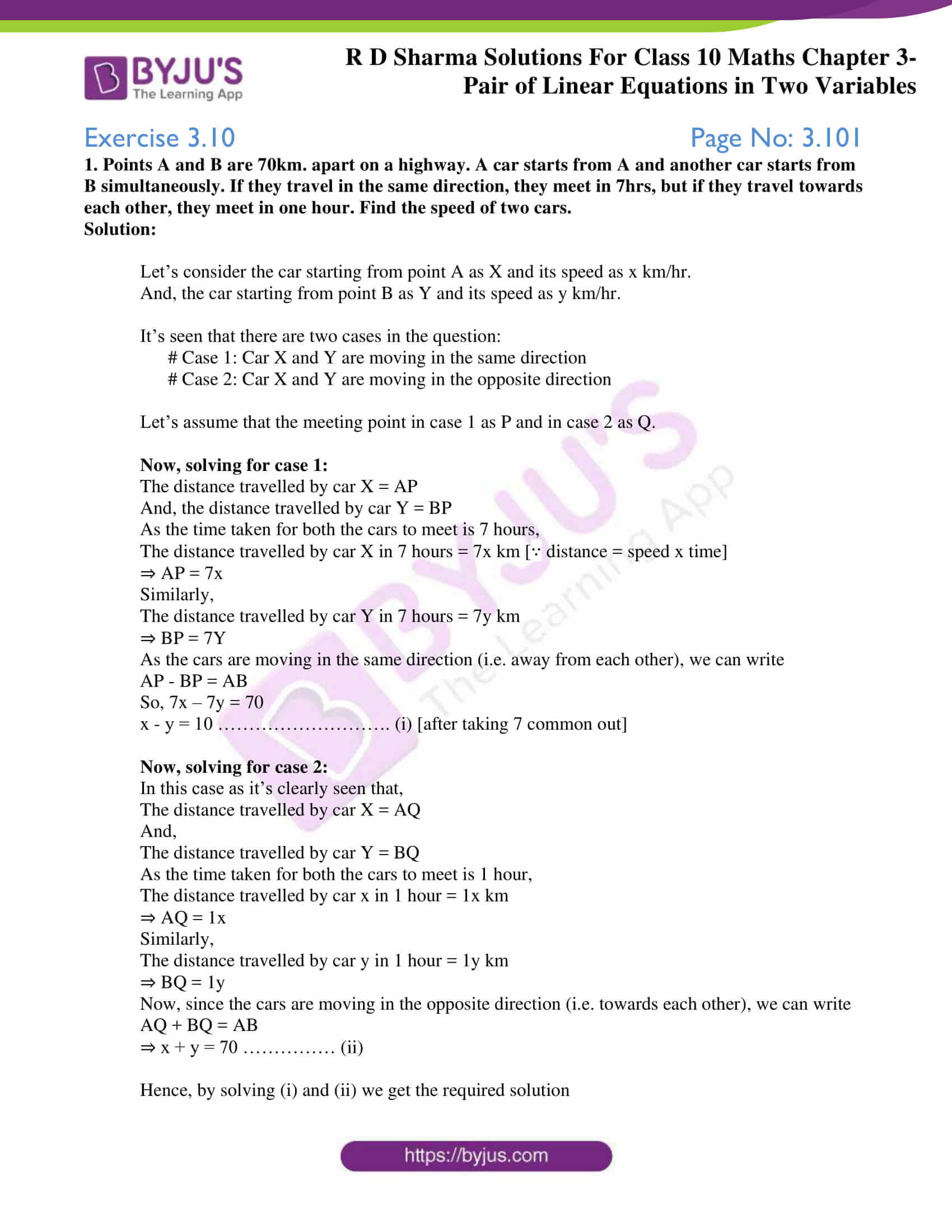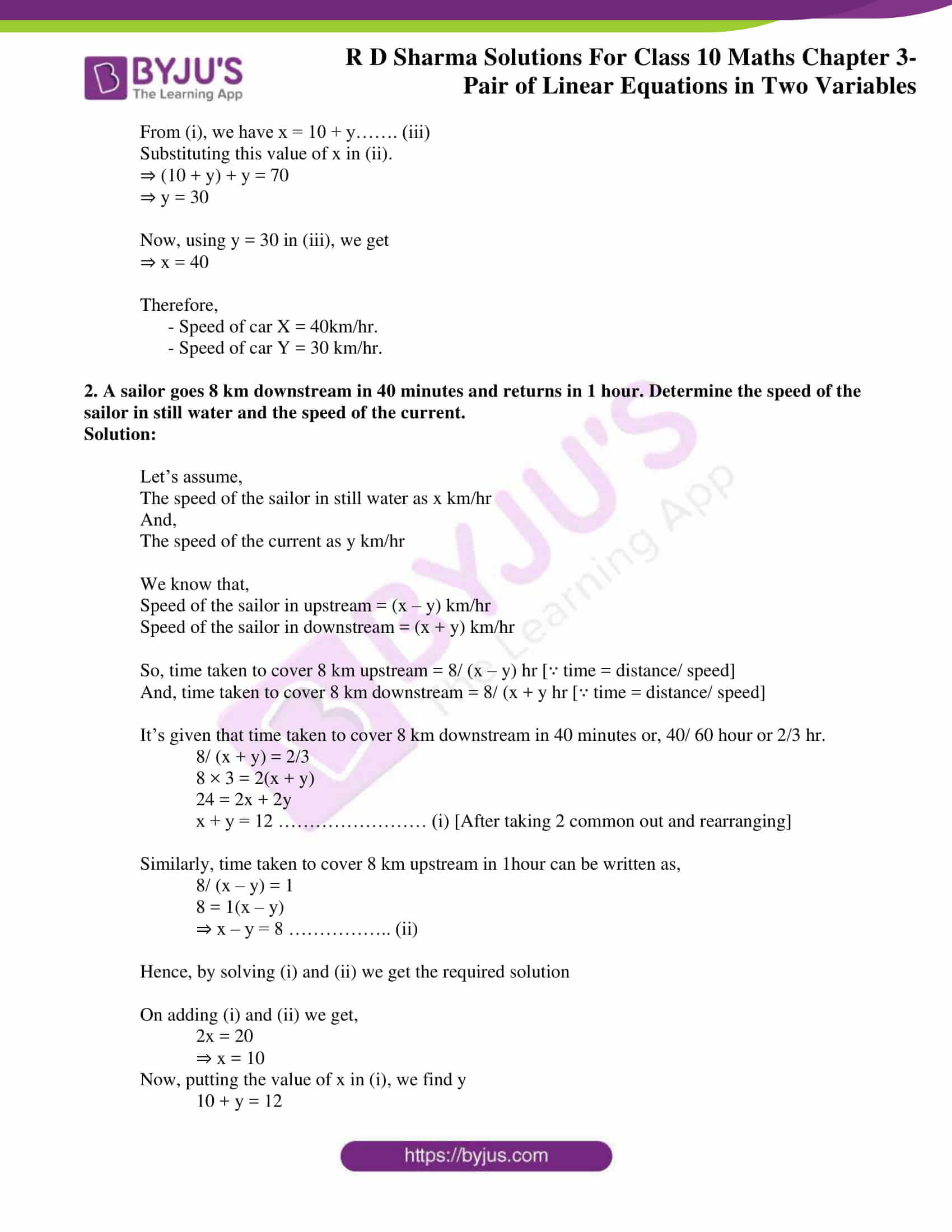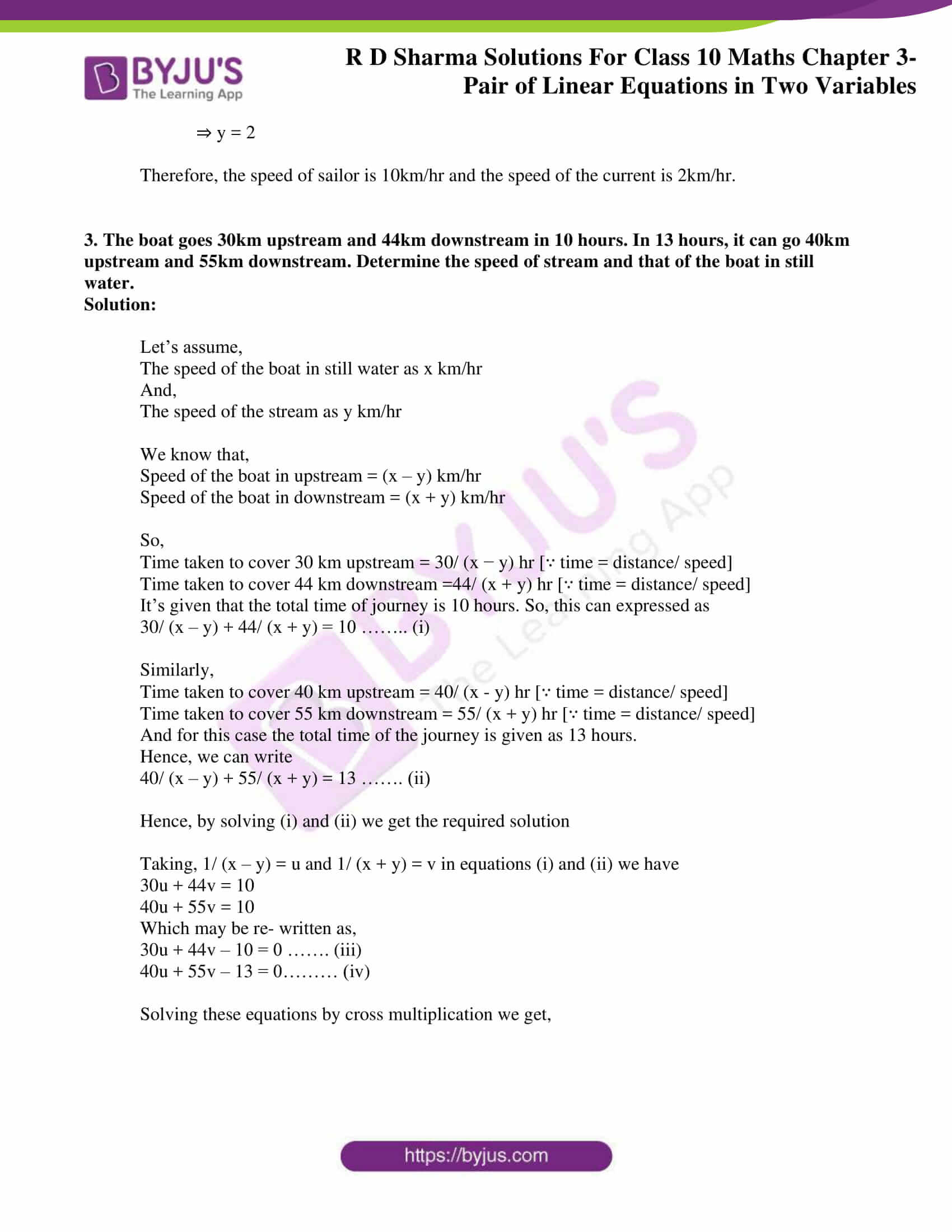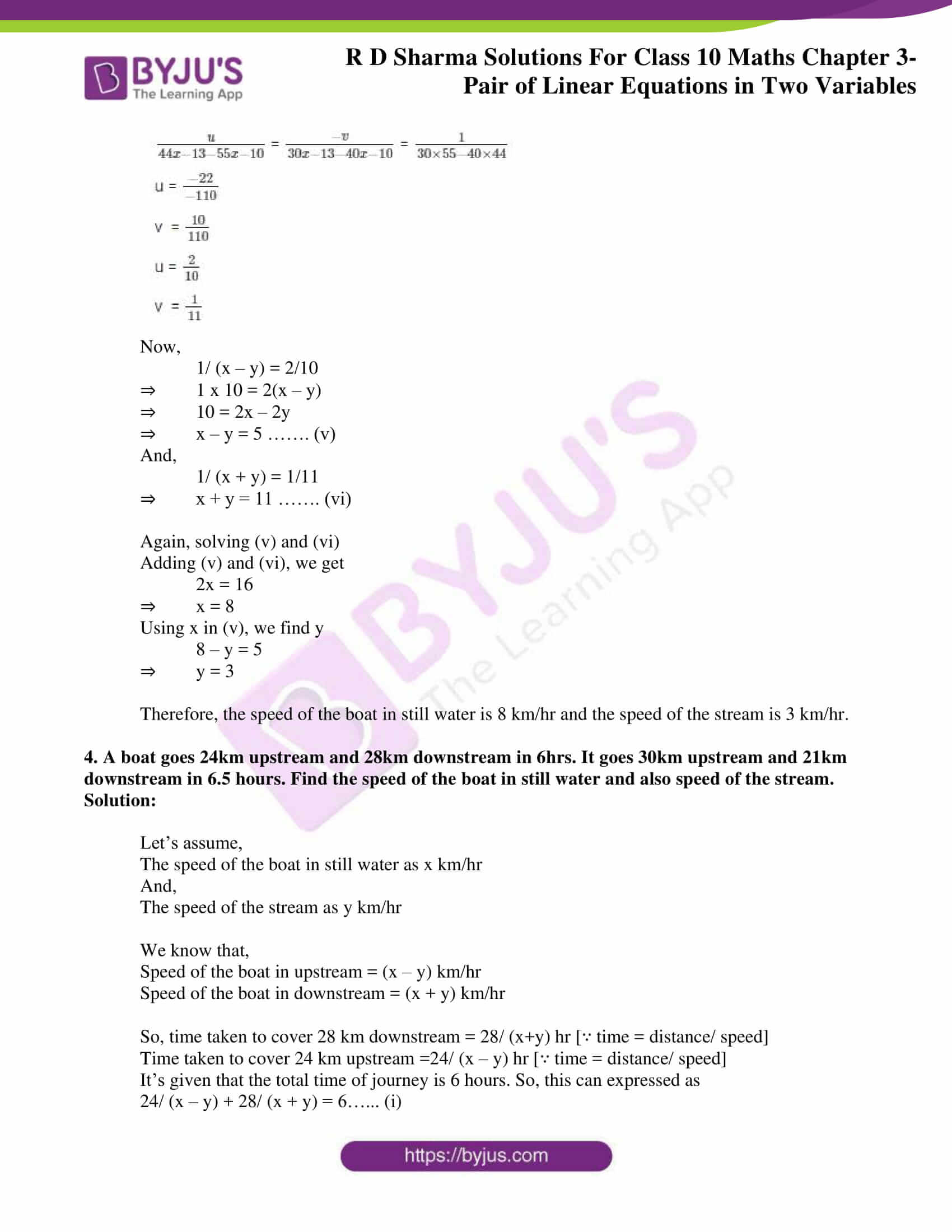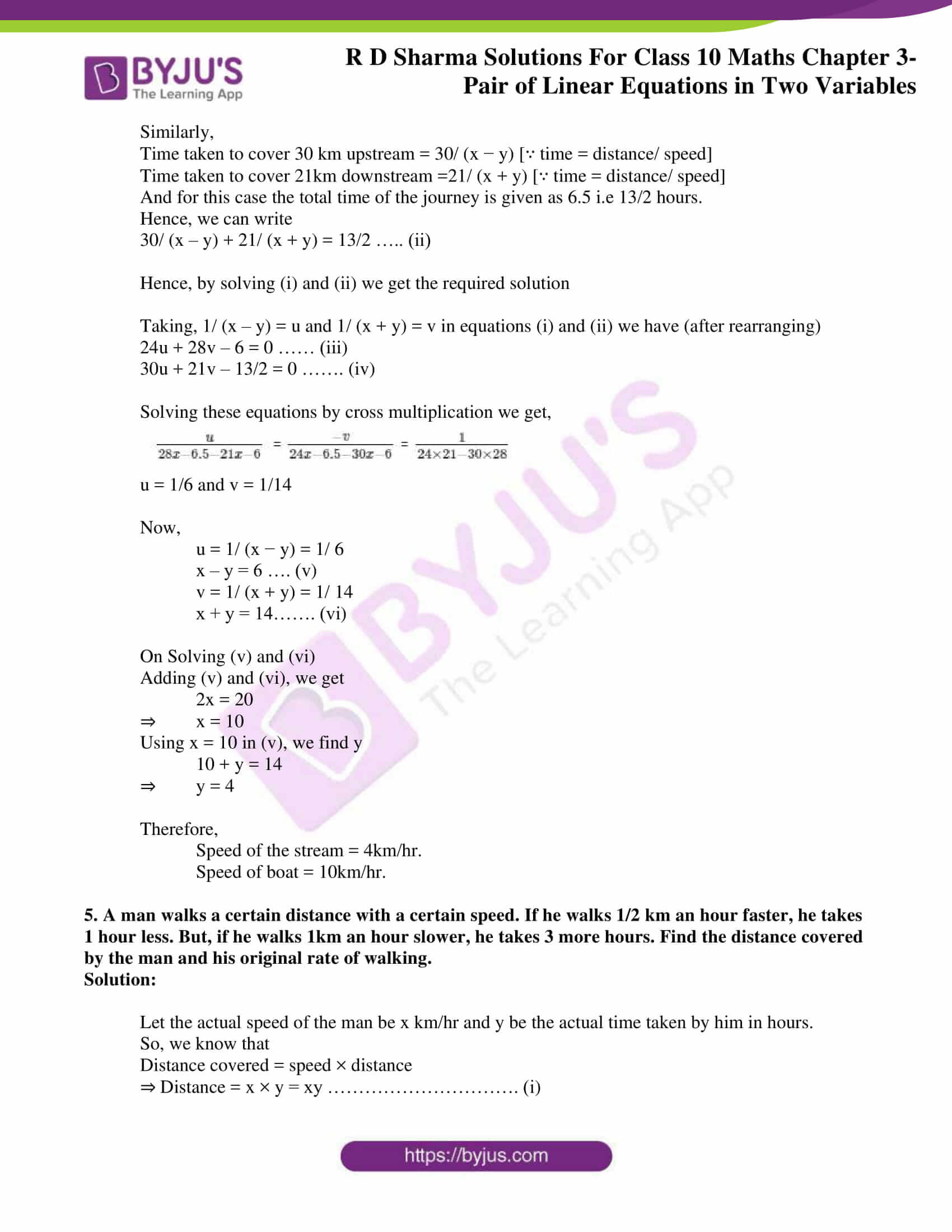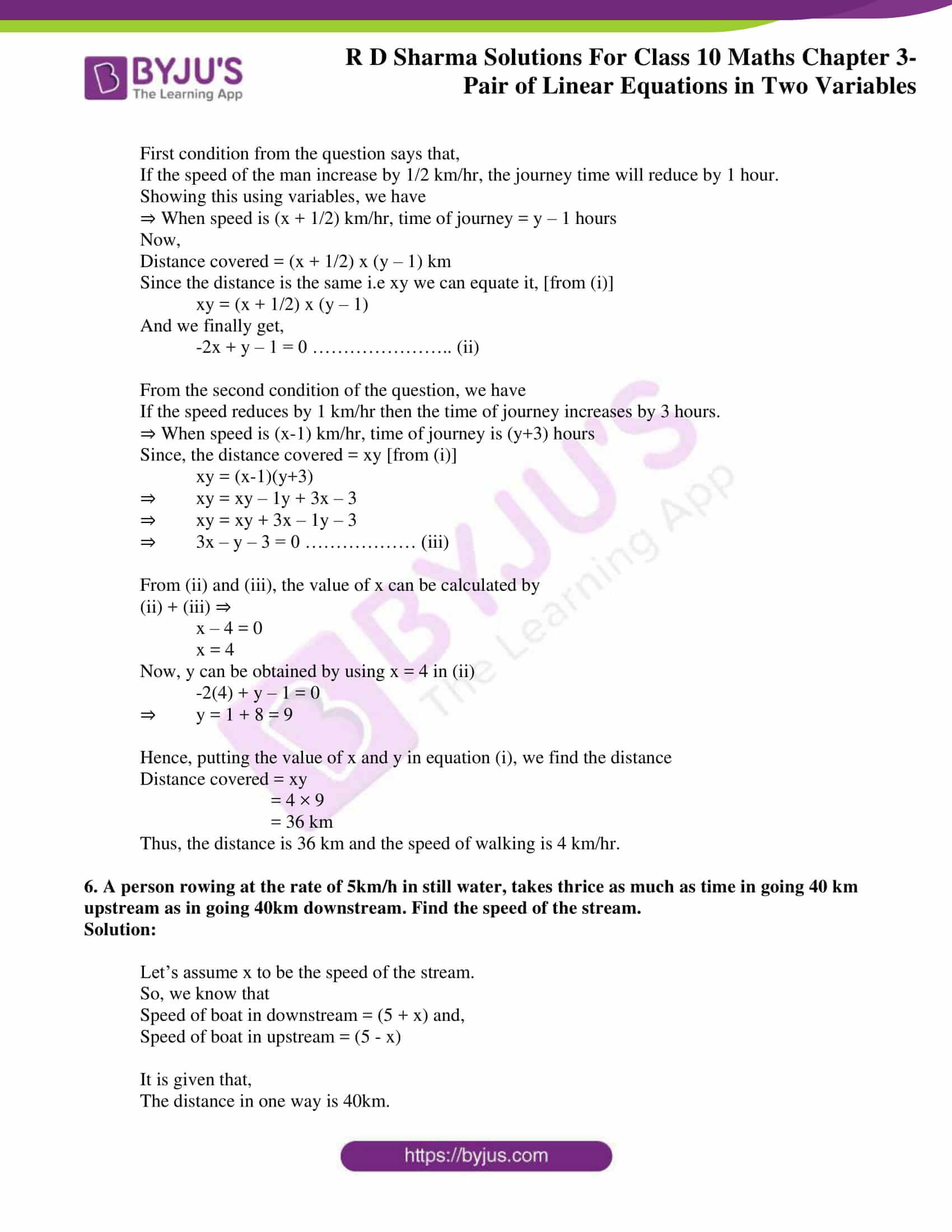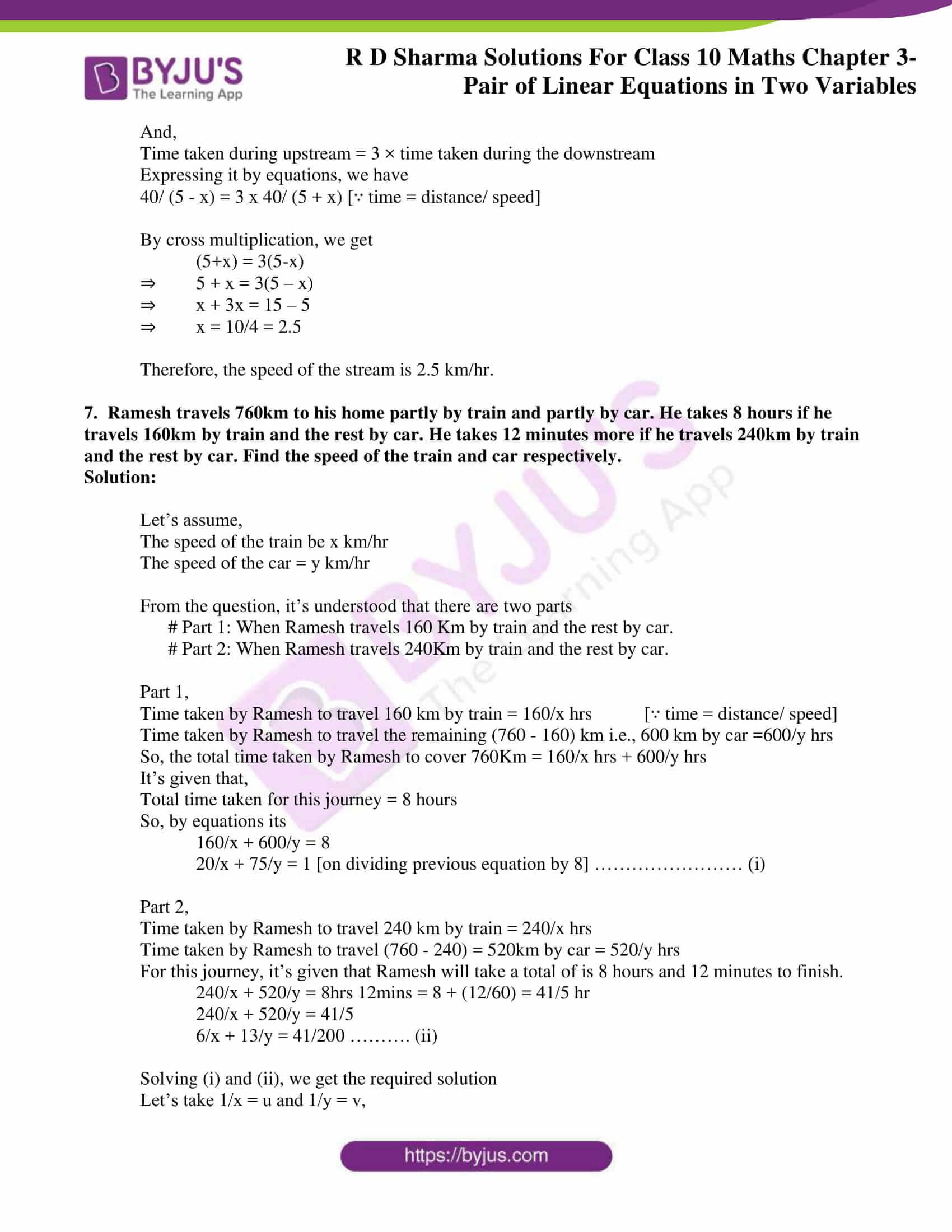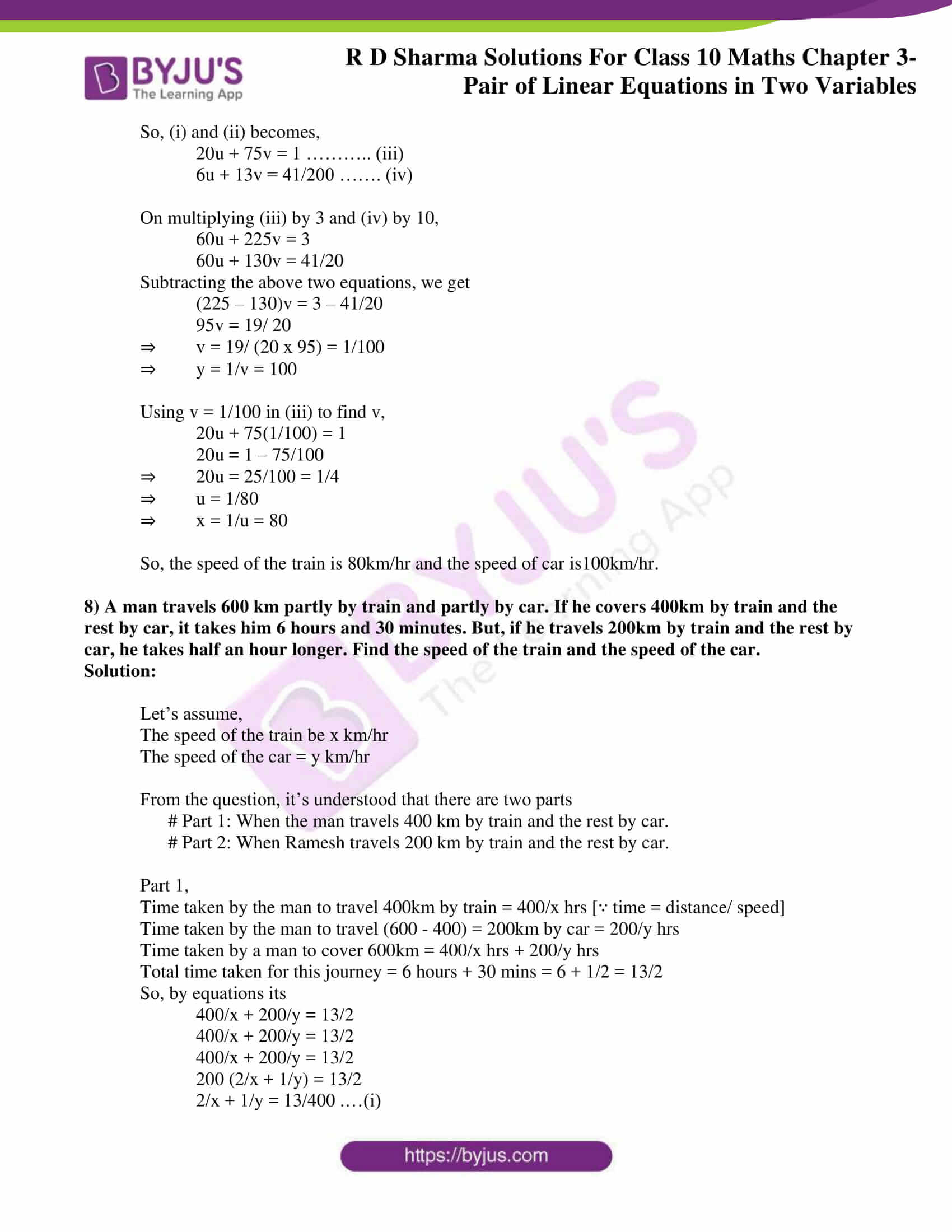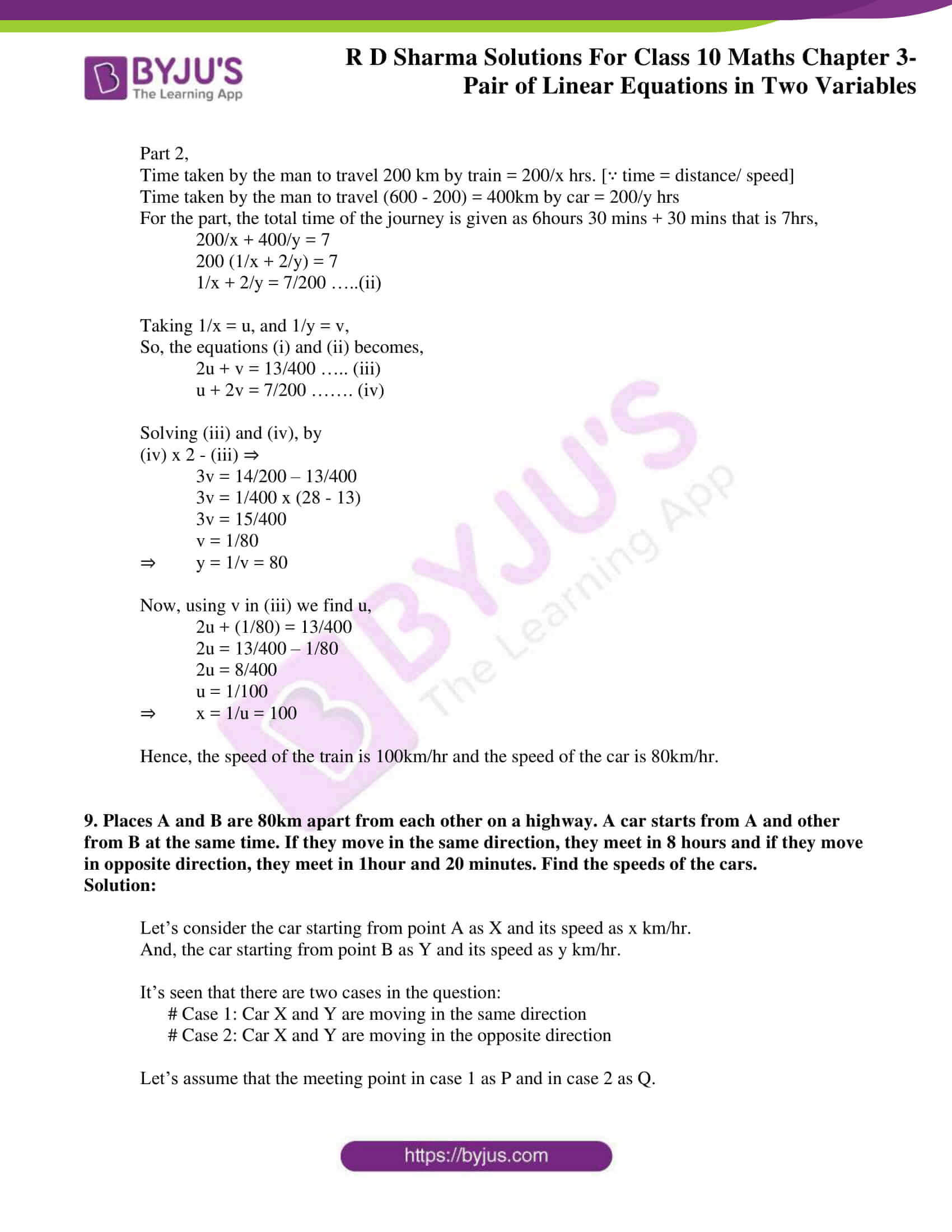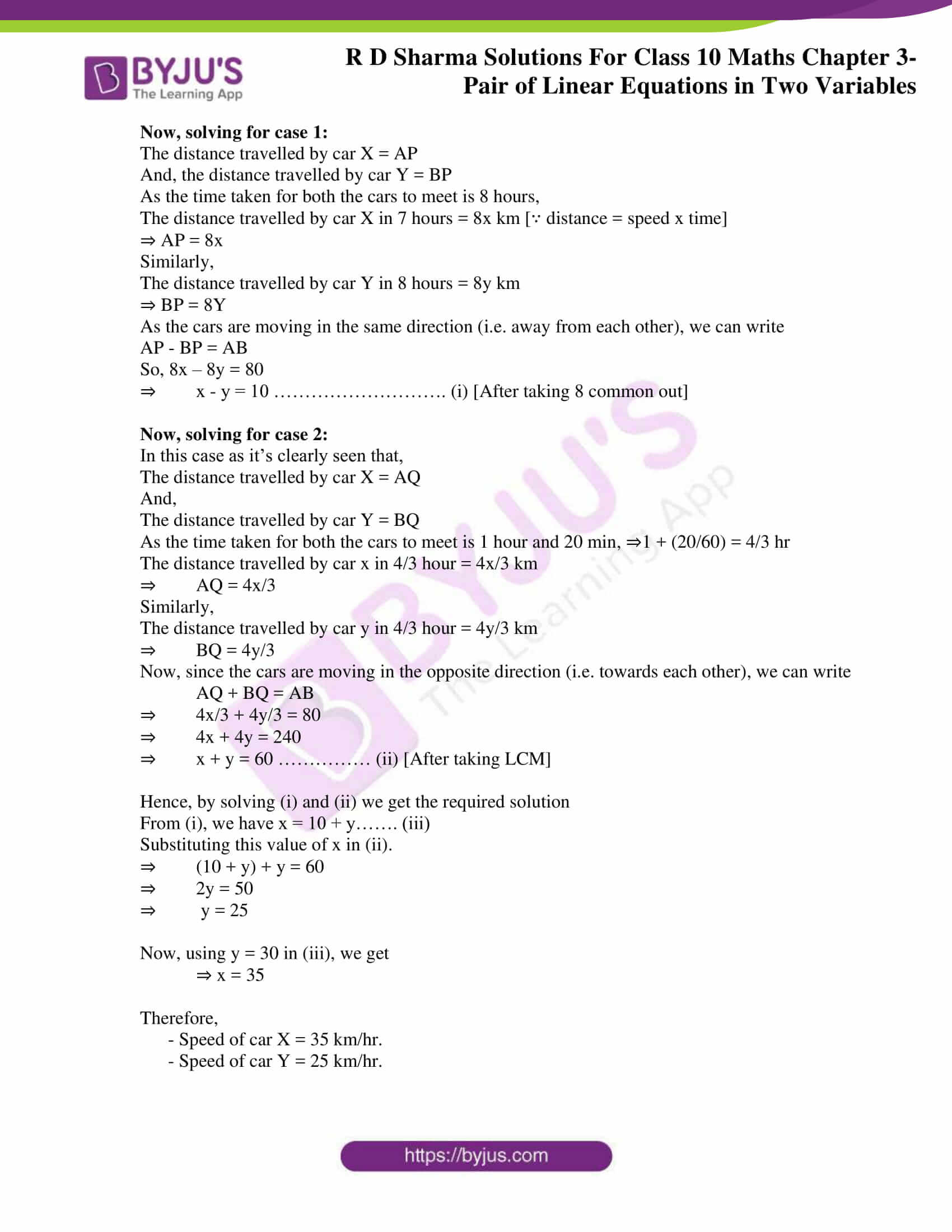### Access RD Sharma Solutions for Class 10 Maths Chapter 3 Pair of Linear Equations In Two Variables Exercise 3.10

1. Points A and B are 70km. apart on a highway. A car starts from A and another car starts from B simultaneously. If they travel in the same direction, they meet in 7hrs, but if they travel towards each other, they meet in one hour. Find the speed of two cars.

Solution:

Let’s consider the car starting from point A as X and its speed as x km/hr.

And, the car starting from point B as Y and its speed as y km/hr.

It’s seen that there are two cases in the question:

# Case 1: Car X and Y are moving in the same direction

# Case 2: Car X and Y are moving in the opposite direction

Let’s assume that the meeting point in case 1 as P and in case 2 as Q.

Now, solving for case 1:

The distance travelled by car X = AP

And, the distance travelled by car Y = BP

As the time taken for both the cars to meet is 7 hours,

The distance travelled by car X in 7 hours = 7x km [∵ distance = speed x time]

⇒ AP = 7x

Similarly,

The distance travelled by car Y in 7 hours = 7y km

⇒ BP = 7Y

As the cars are moving in the same direction (i.e. away from each other), we can write

AP – BP = AB

So, 7x – 7y = 70

x – y = 10 ………………………. (i) [after taking 7 common out]

Now, solving for case 2:

In this case as it’s clearly seen that,

The distance travelled by car X = AQ

And,

The distance travelled by car Y = BQ

As the time taken for both the cars to meet is 1 hour,

The distance travelled by car x in 1 hour = 1x km

⇒ AQ = 1x

Similarly,

The distance travelled by car y in 1 hour = 1y km

⇒ BQ = 1y

Now, since the cars are moving in the opposite direction (i.e. towards each other), we can write

AQ + BQ = AB

⇒ x + y = 70 …………… (ii)

Hence, by solving (i) and (ii) we get the required solution

From (i), we have x = 10 + y……. (iii)

Substituting this value of x in (ii).

⇒ (10 + y) + y = 70

⇒ y = 30

Now, using y = 30 in (iii), we get

⇒ x = 40

Therefore,

– Speed of car X = 40km/hr.

– Speed of car Y = 30 km/hr.

2. A sailor goes 8 km downstream in 40 minutes and returns in 1 hour. Determine the speed of the sailor in still water and the speed of the current.

Solution:

Let’s assume,

The speed of the sailor in still water as x km/hr

And,

The speed of the current as y km/hr

We know that,

Speed of the sailor in upstream = (x – y) km/hr

Speed of the sailor in downstream = (x + y) km/hr

So, time taken to cover 8 km upstream = 8/ (x – y) hr [∵ time = distance/ speed]

And, time taken to cover 8 km downstream = 8/ (x + y hr [∵ time = distance/ speed]

It’s given that time taken to cover 8 km downstream in 40 minutes or, 40/ 60 hour or 2/3 hr.

8/ (x + y) = 2/3

8 × 3 = 2(x + y)

24 = 2x + 2y

x + y = 12 …………………… (i) [After taking 2 common out and rearranging]

Similarly, time taken to cover 8 km upstream in 1hour can be written as,

8/ (x – y) = 1

8 = 1(x – y)

⇒ x – y = 8 …………….. (ii)

Hence, by solving (i) and (ii) we get the required solution

On adding (i) and (ii) we get,

2x = 20

⇒ x = 10

Now, putting the value of x in (i), we find y

10 + y = 12

⇒ y = 2

Therefore, the speed of sailor is 10km/hr and the speed of the current is 2km/hr.

3. The boat goes 30km upstream and 44km downstream in 10 hours. In 13 hours, it can go 40km upstream and 55km downstream. Determine the speed of stream and that of the boat in still water.

Solution:

Let’s assume,

The speed of the boat in still water as x km/hr

And,

The speed of the stream as y km/hr

We know that,

Speed of the boat in upstream = (x – y) km/hr

Speed of the boat in downstream = (x + y) km/hr

So,

Time taken to cover 30 km upstream = 30/ (x − y) hr [∵ time = distance/ speed]

Time taken to cover 44 km downstream =44/ (x + y) hr [∵ time = distance/ speed]

It’s given that the total time of journey is 10 hours. So, this can expressed as

30/ (x – y) + 44/ (x + y) = 10 …….. (i)

Similarly,

Time taken to cover 40 km upstream = 40/ (x – y) hr [∵ time = distance/ speed]

Time taken to cover 55 km downstream = 55/ (x + y) hr [∵ time = distance/ speed]

And for this case the total time of the journey is given as 13 hours.

Hence, we can write

40/ (x – y) + 55/ (x + y) = 13 ……. (ii)

Hence, by solving (i) and (ii) we get the required solution

Taking, 1/ (x – y) = u and 1/ (x + y) = v in equations (i) and (ii) we have

30u + 44v = 10

40u + 55v = 10

Which may be re- written as,

30u + 44v – 10 = 0 ……. (iii)

40u + 55v – 13 = 0……… (iv)

Solving these equations by cross multiplication we get,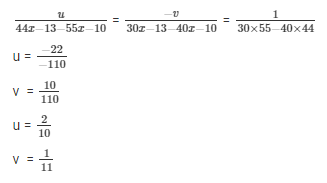Now,

1/ (x – y) = 2/10

⇒ 1 x 10 = 2(x – y)

⇒ 10 = 2x – 2y

⇒ x – y = 5 ……. (v)

And,

1/ (x + y) = 1/11

⇒ x + y = 11 ……. (vi)

Again, solving (v) and (vi)

Adding (v) and (vi), we get

2x = 16

⇒ x = 8

Using x in (v), we find y

8 – y = 5

⇒ y = 3

Therefore, the speed of the boat in still water is 8 km/hr and the speed of the stream is 3 km/hr.

4. A boat goes 24km upstream and 28km downstream in 6hrs. It goes 30km upstream and 21km downstream in 6.5 hours. Find the speed of the boat in still water and also speed of the stream.

Solution:

Let’s assume,

The speed of the boat in still water as x km/hr

And,

The speed of the stream as y km/hr

We know that,

Speed of the boat in upstream = (x – y) km/hr

Speed of the boat in downstream = (x + y) km/hr

So, time taken to cover 28 km downstream = 28/ (x+y) hr [∵ time = distance/ speed]

Time taken to cover 24 km upstream =24/ (x – y) hr [∵ time = distance/ speed]

It’s given that the total time of journey is 6 hours. So, this can expressed as

24/ (x – y) + 28/ (x + y) = 6…… (i)

Similarly,

Time taken to cover 30 km upstream = 30/ (x − y) [∵ time = distance/ speed]

Time taken to cover 21km downstream =21/ (x + y) [∵ time = distance/ speed]

And for this case the total time of the journey is given as 6.5 i.e 13/2 hours.

Hence, we can write

30/ (x – y) + 21/ (x + y) = 13/2 ….. (ii)

Hence, by solving (i) and (ii) we get the required solution

Taking, 1/ (x – y) = u and 1/ (x + y) = v in equations (i) and (ii) we have (after rearranging)

24u + 28v – 6 = 0 …… (iii)

30u + 21v – 13/2 = 0 ……. (iv)

Solving these equations by cross multiplication we get,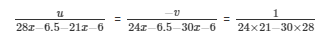u = 1/6 and v = 1/14

Now,

u = 1/ (x − y) = 1/ 6

x – y = 6 …. (v)

v = 1/ (x + y) = 1/ 14

x + y = 14……. (vi)

On Solving (v) and (vi)

Adding (v) and (vi), we get

2x = 20

⇒ x = 10

Using x = 10 in (v), we find y

10 + y = 14

⇒ y = 4

Therefore,

Speed of the stream = 4km/hr.

Speed of boat = 10km/hr.

5. A man walks a certain distance with a certain speed. If he walks 1/2 km an hour faster, he takes 1 hour less. But, if he walks 1km an hour slower, he takes 3 more hours. Find the distance covered by the man and his original rate of walking.

Solution:

Let the actual speed of the man be x km/hr and y be the actual time taken by him in hours.

So, we know that

Distance covered = speed × distance

⇒ Distance = x × y = xy …………………………. (i)

First condition from the question says that,

If the speed of the man increase by 1/2 km/hr, the journey time will reduce by 1 hour.

Showing this using variables, we have

⇒ When speed is (x + 1/2) km/hr, time of journey = y – 1 hours

Now,

Distance covered = (x + 1/2) x (y – 1) km

Since the distance is the same i.e xy we can equate it, [from (i)]

xy = (x + 1/2) x (y – 1)

And we finally get,

-2x + y – 1 = 0 ………………….. (ii)

From the second condition of the question, we have

If the speed reduces by 1 km/hr then the time of journey increases by 3 hours.

⇒ When speed is (x-1) km/hr, time of journey is (y+3) hours

Since, the distance covered = xy [from (i)]

xy = (x-1)(y+3)

⇒ xy = xy – 1y + 3x – 3

⇒ xy = xy + 3x – 1y – 3

⇒ 3x – y – 3 = 0 ……………… (iii)

From (ii) and (iii), the value of x can be calculated by

(ii) + (iii) ⇒

x – 4 = 0

x = 4

Now, y can be obtained by using x = 4 in (ii)

-2(4) + y – 1 = 0

⇒ y = 1 + 8 = 9

Hence, putting the value of x and y in equation (i), we find the distance

Distance covered = xy

= 4 × 9

= 36 km

Thus, the distance is 36 km and the speed of walking is 4 km/hr.

6. A person rowing at the rate of 5km/h in still water, takes thrice as much as time in going 40 km upstream as in going 40km downstream. Find the speed of the stream.

Solution:

Let’s assume x to be the speed of the stream.

So, we know that

Speed of boat in downstream = (5 + x) and,

Speed of boat in upstream = (5 – x)

It is given that,

The distance in one way is 40km.

And,

Time taken during upstream = 3 × time taken during the downstream

Expressing it by equations, we have

40/ (5 – x) = 3 x 40/ (5 + x) [∵ time = distance/ speed]

By cross multiplication, we get

(5+x) = 3(5-x)

⇒ 5 + x = 3(5 – x)

⇒ x + 3x = 15 – 5

⇒ x = 10/4 = 2.5

Therefore, the speed of the stream is 2.5 km/hr.

7. Ramesh travels 760km to his home partly by train and partly by car. He takes 8 hours if he travels 160km by train and the rest by car. He takes 12 minutes more if he travels 240km by train and the rest by car. Find the speed of the train and car respectively.

Solution:

Let’s assume,

The speed of the train be x km/hr

The speed of the car = y km/hr

From the question, it’s understood that there are two parts

# Part 1: When Ramesh travels 160 Km by train and the rest by car.

# Part 2: When Ramesh travels 240Km by train and the rest by car.

Part 1,

Time taken by Ramesh to travel 160 km by train = 160/x hrs [∵ time = distance/ speed]

Time taken by Ramesh to travel the remaining (760 – 160) km i.e., 600 km by car =600/y hrs

So, the total time taken by Ramesh to cover 760Km = 160/x hrs + 600/y hrs

It’s given that,

Total time taken for this journey = 8 hours

So, by equations its

160/x + 600/y = 8

20/x + 75/y = 1 [on dividing previous equation by 8] …………………… (i)

Part 2,

Time taken by Ramesh to travel 240 km by train = 240/x hrs

Time taken by Ramesh to travel (760 – 240) = 520km by car = 520/y hrs

For this journey, it’s given that Ramesh will take a total of is 8 hours and 12 minutes to finish.

240/x + 520/y = 8hrs 12mins = 8 + (12/60) = 41/5 hr

240/x + 520/y = 41/5

6/x + 13/y = 41/200 ………. (ii)

Solving (i) and (ii), we get the required solution

Let’s take 1/x = u and 1/y = v,

So, (i) and (ii) becomes,

20u + 75v = 1 ……….. (iii)

6u + 13v = 41/200 ……. (iv)

On multiplying (iii) by 3 and (iv) by 10,

60u + 225v = 3

60u + 130v = 41/20

Subtracting the above two equations, we get

(225 – 130)v = 3 – 41/20

95v = 19/ 20

⇒ v = 19/ (20 x 95) = 1/100

⇒ y = 1/v = 100

Using v = 1/100 in (iii) to find v,

20u + 75(1/100) = 1

20u = 1 – 75/100

⇒ 20u = 25/100 = 1/4

⇒ u = 1/80

⇒ x = 1/u = 80

So, the speed of the train is 80km/hr and the speed of car is100km/hr.

8) A man travels 600 km partly by train and partly by car. If he covers 400km by train and the rest by car, it takes him 6 hours and 30 minutes. But, if he travels 200km by train and the rest by car, he takes half an hour longer. Find the speed of the train and the speed of the car.

Solution:

Let’s assume,

The speed of the train be x km/hr

The speed of the car = y km/hr

From the question, it’s understood that there are two parts

# Part 1: When the man travels 400 km by train and the rest by car.

# Part 2: When Ramesh travels 200 km by train and the rest by car.

Part 1,

Time taken by the man to travel 400km by train = 400/x hrs [∵ time = distance/ speed]

Time taken by the man to travel (600 – 400) = 200km by car = 200/y hrs

Time taken by a man to cover 600km = 400/x hrs + 200/y hrs

Total time taken for this journey = 6 hours + 30 mins = 6 + 1/2 = 13/2

So, by equations its

400/x + 200/y = 13/2

400/x + 200/y = 13/2

400/x + 200/y = 13/2

200 (2/x + 1/y) = 13/2

2/x + 1/y = 13/400 .…(i)

Part 2,

Time taken by the man to travel 200 km by train = 200/x hrs. [∵ time = distance/ speed]

Time taken by the man to travel (600 – 200) = 400km by car = 200/y hrs

For the part, the total time of the journey is given as 6hours 30 mins + 30 mins that is 7hrs,

200/x + 400/y = 7

200 (1/x + 2/y) = 7

1/x + 2/y = 7/200 …..(ii)

Taking 1/x = u, and 1/y = v,

So, the equations (i) and (ii) becomes,

2u + v = 13/400 ….. (iii)

u + 2v = 7/200 ……. (iv)

Solving (iii) and (iv), by

(iv) x 2 – (iii) ⇒

3v = 14/200 – 13/400

3v = 1/400 x (28 – 13)

3v = 15/400

v = 1/80

⇒ y = 1/v = 80

Now, using v in (iii) we find u,

2u + (1/80) = 13/400

2u = 13/400 – 1/80

2u = 8/400

u = 1/100

⇒ x = 1/u = 100

Hence, the speed of the train is 100km/hr and the speed of the car is 80km/hr.

9. Places A and B are 80km apart from each other on a highway. A car starts from A and other from B at the same time. If they move in the same direction, they meet in 8 hours and if they move in opposite direction, they meet in 1hour and 20 minutes. Find the speeds of the cars.

Solution:

Let’s consider the car starting from point A as X and its speed as x km/hr.

And, the car starting from point B as Y and its speed as y km/hr.

It’s seen that there are two cases in the question:

# Case 1: Car X and Y are moving in the same direction

# Case 2: Car X and Y are moving in the opposite direction

Let’s assume that the meeting point in case 1 as P and in case 2 as Q.

Now, solving for case 1:

The distance travelled by car X = AP

And, the distance travelled by car Y = BP

As the time taken for both the cars to meet is 8 hours,

The distance travelled by car X in 7 hours = 8x km [∵ distance = speed x time]

⇒ AP = 8x

Similarly,

The distance travelled by car Y in 8 hours = 8y km

⇒ BP = 8Y

As the cars are moving in the same direction (i.e. away from each other), we can write

AP – BP = AB

So, 8x – 8y = 80

⇒ x – y = 10 ………………………. (i) [After taking 8 common out]

Now, solving for case 2:

In this case as it’s clearly seen that,

The distance travelled by car X = AQ

And,

The distance travelled by car Y = BQ

As the time taken for both the cars to meet is 1 hour and 20 min, ⇒1 + (20/60) = 4/3 hr

The distance travelled by car x in 4/3 hour = 4x/3 km

⇒ AQ = 4x/3

Similarly,

The distance travelled by car y in 4/3 hour = 4y/3 km

⇒ BQ = 4y/3

Now, since the cars are moving in the opposite direction (i.e. towards each other), we can write

AQ + BQ = AB

⇒ 4x/3 + 4y/3 = 80

⇒ 4x + 4y = 240

⇒ x + y = 60 …………… (ii) [After taking LCM]

Hence, by solving (i) and (ii) we get the required solution

From (i), we have x = 10 + y……. (iii)

Substituting this value of x in (ii).

⇒ (10 + y) + y = 60

⇒ 2y = 50

⇒ y = 25

Now, using y = 30 in (iii), we get

⇒ x = 35

Therefore,

– Speed of car X = 35 km/hr.

– Speed of car Y = 25 km/hr.